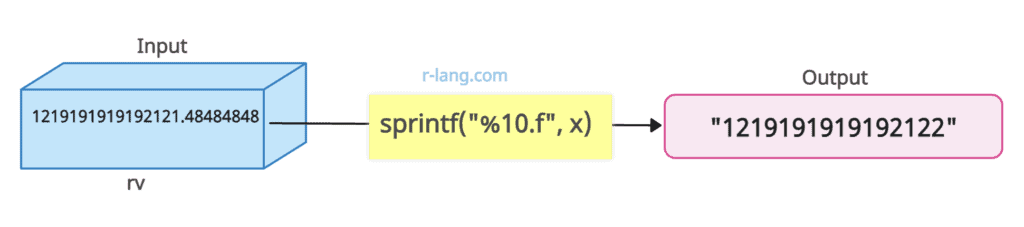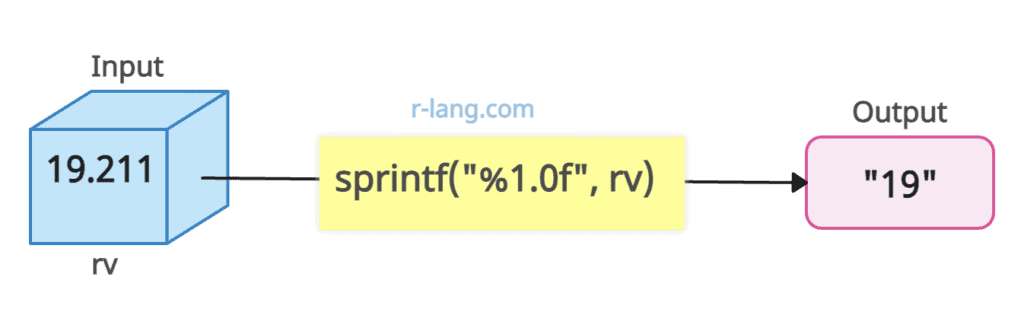# How to Use sprintf() Function in R

The sprintf() function in R is used to print the formatted strings. For example, use the sprintf(“%f”, x) function to format a numeric value x with six digits after the decimal point.

## Syntax

``sprintf(fmt, x)``

## Parameters

1. fmt: It is a character vector of format strings, each of up to 8192 bytes.
2. x: It is the value to format.

## Return Value

It returns the character vector of length that of the longest input.

## Example 1: Format decimal places

The above figure shows that the sprintf() function is used to format and display the value of x with only 2 digits after the decimal point. Let’s implement it into the program.

``````# Define value
x <- 21.41347382

# Only display 2 digits after decimal place
sprintf("%.2f", x)
``````

Output

`` "21.41"``

## Example 2: Format digits before decimal pointFigure 2: Use sprintf() function to format digits before decimal point

We used the sprintf() function to format and display the value of x as a floating-point number with no digits after the decimal point while ensuring a minimum width of 10 characters for the output.

``````# Define value
x <- 1219191919192121.48484848

sprintf("%10.f", x)``````

Output

`````` "1219191919192122"
``````

## Example 3: Format value using Scientific Notation

``````rv <- 19.211

sprintf("%e", rv)``````

You can return the upper case E to the RStudio console.

``````data <- 19.211

sprintf("%E", data)``````

Output

`` "1.921100E+01"``

## Example 4: Format digits before the decimal pointFigure 4: Format digits before the decimal point

``````rv <- 19.211

sprintf("%1.0f", rv)``````

Output

`` "19"``

## Example 5: Format Digits After Decimal Point

``````data <- 21.19461118

#only display 2 digits after decimal place
sprintf("%.2f", data)``````

Output

`` "21.19"``

## Example 6: Format a value in String

``````data <- 21.19461118

sprintf("I am away %.1f miles from Perth city", data)``````

Output

`` "I am away 21.2 miles from Perth city"``

You can also format multiple values in a string:

``````x1 <- 21.11
x2 <- 11.21

sprintf("I rode my car %.1f miles and then ran %.2f miles", x1, x2)``````

Output

`` "I rode my car 21.1 miles and then ran 11.21 miles"``

That’s it.